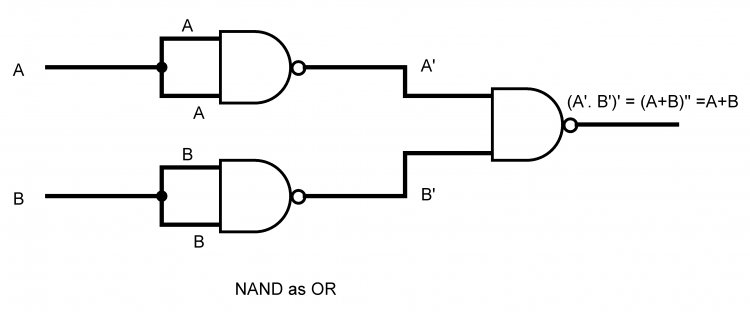# Combination Logic Design

## A complete notes on Combinational Logic Design for BCA and BIT students. Topics: Basis gates,AND gate,OR gate,NOT gate,universal gates,NOR gate,NAND gate,XOR gate,NOT gate using NOR and NAND gate,OR gate using NOR and NAND gate,AND gate using NOR and NAND gate,different laws for gates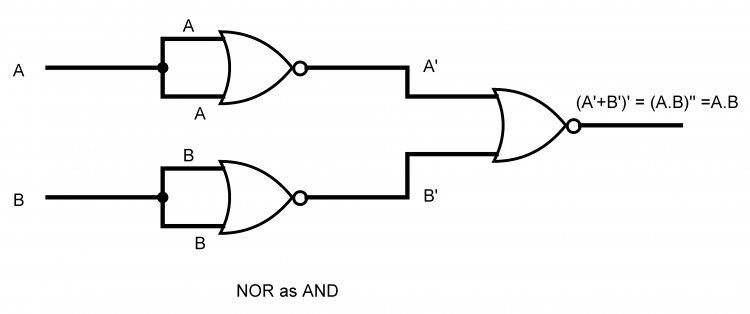3.1 Basic Logic Gates AND, OR and NOT

Logic gates are building block of any digital system. It is an electronic circuit having one or more than one inputs and only one output.

Logic means certain declarative statement which is true if certain conditions are fulfilled and false if that condition is not meet.

Boolean algebra is the algebra of logic, which deals with the study or binary variables and logical operations.

Boolean Variable:

A computer is a binary digital system. It has two possible states: High or 1 and Low or 0. A variable signal changes its state with time. Its value may be 1 at some point and 0 on another. Thus the variables that have only two values 1 and 0 are called Boolean variables or logic variables.

They are denoted by A, B, X, Y…..

Logic function(Boolean function):

A logic function is an expression formed by binary variable, binary operators OR, AND , unary operator NOT, parenthesis, and equal sign.
E.g. of logic function

F = X.Y.Z’ + X.Y where, X, Y, Z are Boolean Variables

The right hand side of above equation is called as expression. Each occurrence of a variable or its complement in an expression is called literal.

Here, there are three variables(X, Y and Z) and five literals (X, Y, Z’, X and Y).

Truth Table:

A table which represents the input-output relationship of the binary variables for each logical gate is called truth table. It shows the relation between all inputs and output in tabular form.

Thus a truth table is a table representing the results of the logical operations on all possible combinations of logical values. The number of rows in a truth table is equal to 2n (where n=no. of variables).

For e.g. for 3 input variables, no. of rows will be 23 = 8.

E.g.

 A B C 0 0 0 0 0 1 0 1 0 0 1 1 1 0 0 1 0 1 1 1 0 1 1 1

Boolean Operators and operands:

Operation can be defined as the action upon the data and operator signifies the operation. Operator is the symbol that defines the operation.

The basic operator used in Boolean algebra are AND, OR and NOT.

Every operation can be expressed in terms of these basic operators.

AND OPERATOR

Represented by “.”. So, A AND B can be represented as A.B

The result of AND operation is exactly same as simple arithmetic multiplication. The result will only be true (1) when all the inputs are true.

OR OPERATOR

Represented by “+”. So, A OR B can be represented as A+B.

The result is not exactly same as those of arithmetic addition. In this operation the result is true(1), if at least one input is true.

Operands

Operands are the data items on which the operation is performed. In operation A+B, A and B are the operands. The value of operands A and B change to either 0 and 1.

Logic Gates

A logic gate is an electronic circuit that operates on one or more input signals to produce an output signal. The logic gate is used for binary operation and is the basic component of digital computer. The function of gate is expressed by the means of an algebraic expression.

The basic gates in a digital computer are:

• AND gate
• OR gate
• NOT gate

AND gate

• It performs logical multiplication on its input.
• The output is high if and only if all the inputs to the gate are high(1). Otherwise, the output will be low(0).
• The algebraic expression is F = A . B, where ‘ . ’ stands for AND operation , A and B are inputs and F is output.
• The graph and truth table is given as
 Inputs Output A B A.B(AND OPERATION) 0 0 0 0 1 0 1 0 0 1 1 1

OR gate:

• It performs the logical addition on its input.
• The output will be high if anyone input is high.
• The output will be low if and only if all inputs are simultaneously low.
• The algebraic expression is F = A + B
 Inputs Outputs A B A+B(OR Operation) 0 0 0 0 1 1 1 0 1 1 1 1

NOT gate:

• It has one input and one output .
• It is also called inverter because its output is the inverted version of its input.
• The algebraic expression is F = A’ where, A is input and F is output.
 Input Output A A'(NOT Operation) 0 1 1 0

Apart from the basic gates, there are other gates derived from basic gates, which are:

• NAND gate
• NOR gate
• Exclusive OR (XOR) gate
• Exclusive NOR (XNOR) gate

3.2 Universal Logic Gates NAND, NOR

NAND gate

• It is combination of an AND gate and a NOT gate.
• A NAND gate is equivalent to an AND gate followed by an inverter.
• It produces low(0) output, when all inputs are high(1). Otherwise, the output is high if one or more inputs are low.
• Algebraic expression F = (A.B)’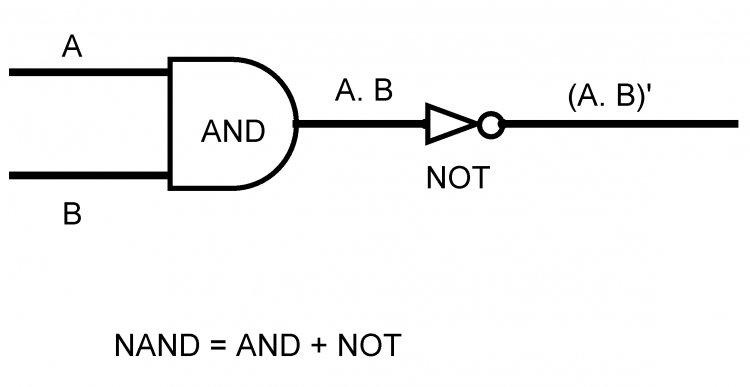Inputs Output A B (A.B)' 0 0 1 0 1 1 1 0 1 1 1 0

NOR gate:

• It is combination of an OR gate and a NOT gate.
• A NOR gate is equivalent to an OR gate followed by an inverter.
• This gate produce high(1) output when all inputs are low(0). Otherwise, The output is low(0)
• Algebraic expression  F = ( A + B)’ Where, A and B are inputs and F is output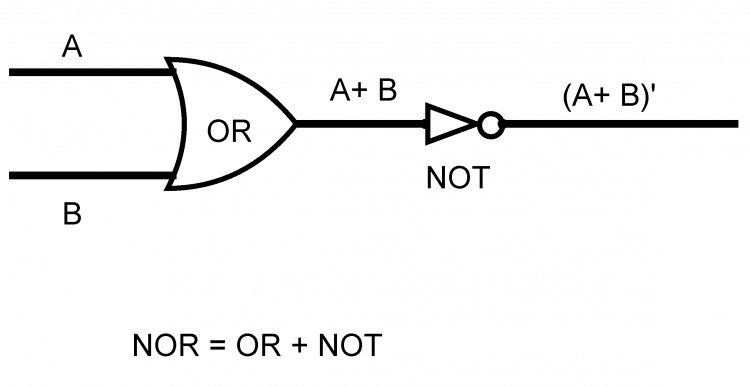Inputs Output A B (A+B)' 0 0 1 0 1 0 1 0 0 1 1 0

Derived gate(special purpose gate)

1.Exclusive OR gate(X-OR) gate:

The output is high if inputs are different and low if both inputs are same.

The algebraic function is F =A ⨁ B = A’B + AB’

Exclusive NOR gate(X-NOR):

• XNOR gate is equivalent to an XOR gate followed by an inverter.
• The output is high(1) if both inputs are same and low(0) if inputs are different.
• The algebraic function is Y = AB + A’B’ = (A⨀B) = (A⨁ B)’

3.4 Boolean Algebra

Boolean Algebra is algebra of logics, which deals with the study of binary variables and logic operations. It is a two valued system of algebra that represents logical relationships and operations. The two values are 1(true) and 0(false).

Boolean algebra is used to analyze and simplify the digital circuits.

The rules of Boolean algebra are different from those of conventional algebra in the following manners:

1. Symbols used in Boolean algebra do not represent numerical values
2. Arithmetic operations like subtraction, division are not performed.
3. There is no fractions, negative numbers, squares, imaginary numbers etc.
4. Boolean algebra allows only two possible values (0 and 1) for any variables.

Boolean Postulates:

Boolean postulates are the fundamental conditions or self-evident proposition(A proposition is a statement that can be either true or false).

The Boolean postulates originate from basic logic operations- AND, OR and NOT. These postulates defines the operations.

Law of Boolean Algebra(Boolean Theorems)

1. Commutative Law:

This law allows change In the position of the input variables in OR and AND expressions.

A + B = B + A

A.B = B.A

1. Associative Law:

This law states if make no difference in what order variables are grouped when OR ing or AND ing more than two variables.

A + (B+C) = (A+B) +C

A.(B.C) = (A.B).C

1. Distributive Law:

This law allows factoring or multiplying out an expression.

A(B+C) = AB + AC

A+(BC) = (A+B).(A+C)

1. Identity Law:

A + 0 = A            A.1 = A

1. Complement Law:

A + A’ = 1

A.A’ = 0

1. Boundedness Law:

A + 1 = 1     A.0 = 0

1. Idempotent Law:

A + A= A  A.A = A

1. Inversion Law:

(A’)’ = A

Show that NOR and NAND gates are universal gates.

NOR and NAND gates are called universal gates because basic gates can be derived from these two gates.

Universal Nature of NOR gate:

1. NOR gate as NOT gate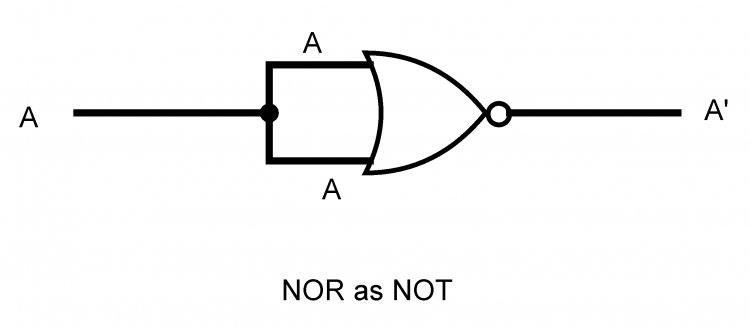2. NOR gate as OR gate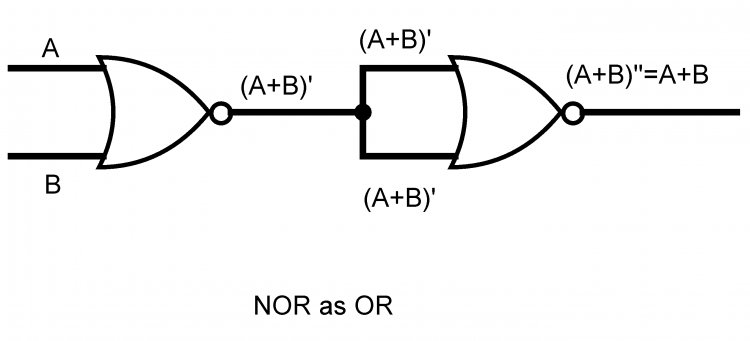3. NOR gate as ANDUniversal Nature of NAND gate:

1. NAND as NOT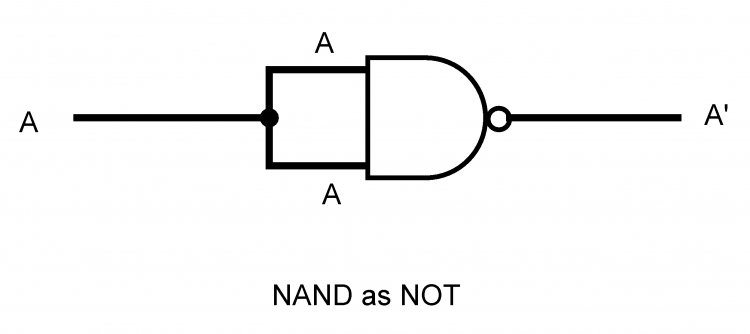2. NAND as AND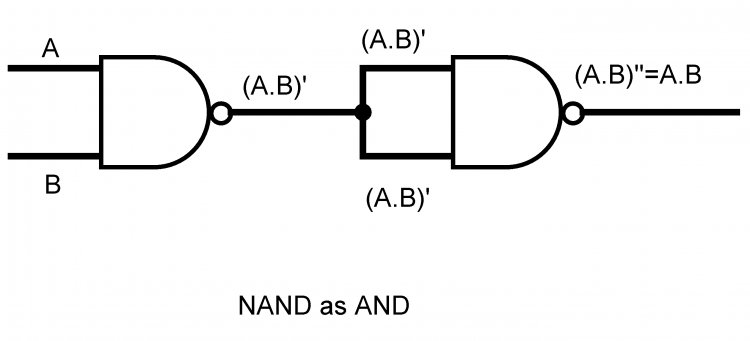3. NAND as OR